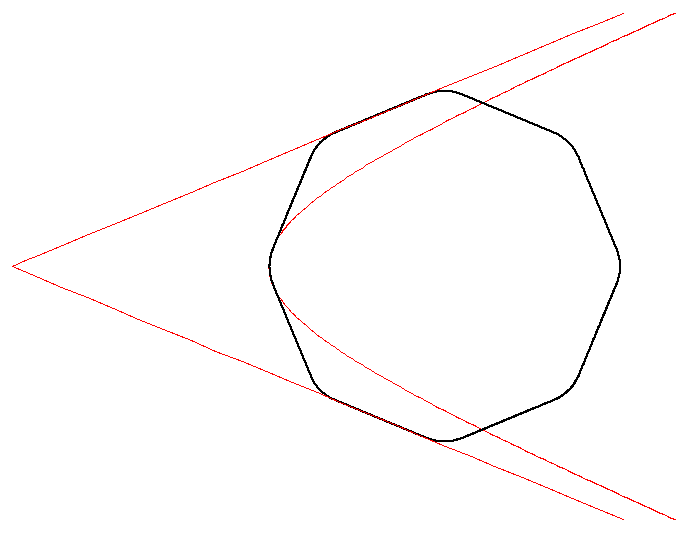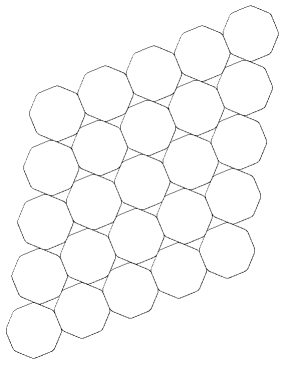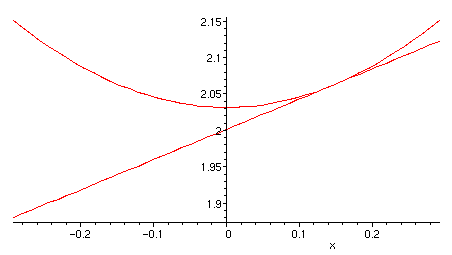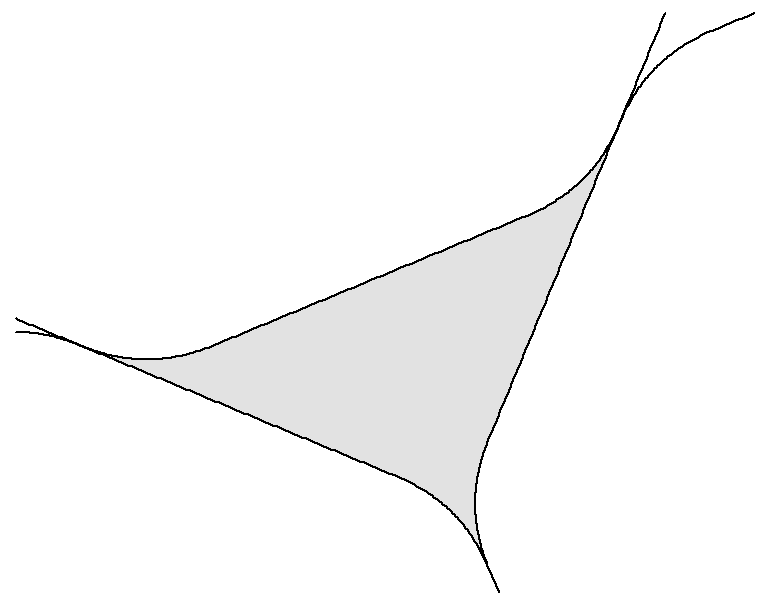LINKS Fachbereich Mathematik UNIX-AG UNI-GH Siegen

# The thinnest densest two-dimensional packing ??

On this page I consider the problem of finding the centraly symmetric convex area whose densest packing is the thinnest. Reinhardt and Mahler describe in their works a possible candidate for this problem: The smoothed octagon. It is obtained by taking a regular octagon and smoothing the edges with a hyperbola which touches the adjecent edges of the octagon and which has the edges adjacent to these edges as asymptotes. (see L.F. Toth, Lagerungen in der Ebene auf der Kugel und im Raum p. 104)The equation which describes the hyperbola is l²x²-m²=y², with l=sqrt(2)-1, m=sqrt(6*sqrt(2)-8)*(sqrt(2)+1)/2. The edges of the octagon touched by the hyperbola lie on y=±(sqrt(2)+1)*(x-2), with x›=2. The asymptotes of the hyperbola are y=±l*x.
The densest packing of this area is the following:To every octagon, there are two holes. If the packing contains regular octagons of edgelength e, the area of a hole, with two edges of length e/sqrt(2) and one of length e, is e²/4. The area of the octagon with edgelength e is 2e²/(sqrt(2)-1). The density is now simple to compute. One gets for the density: 4/(3+sqrt(2)) = 0.90616... . If you consider the smoothed octagon, you get for the density (for example by integrating the formula for the hyperbola. See following picture): (9 - sqrt(32) - ln(2))/(sqrt(8)-1) = 0.90241... . Probably this is the thinnest tightest two-dimensional Packing and the density of the densest packing of any convex area is greater or equal than this value.A closer look at a hole of this packing:Peter Scholl (Peter.Scholl@unix-ag.org)